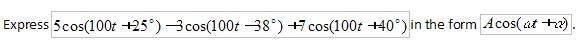# Simplify complex number expression into acos(wt+x)

Gold Member

## Homework Statement

I could not type it here, so i made a screenshot and posted it below.## Homework Equations

it's about complex numbers undergraduate level. i'm currently doing Euler's formula and De Moivre's theorem, although i'm not sure that the solution lies there.

## The Attempt at a Solution

i tried doing it by adding the values inside the cos() but when verifying via calculator with a dummy data, it didn't add up.

tiny-tim
Homework Helper
hi sharks!try expaning each cos into the form Acos100t + Bsin100tGold Member
Hi tiny-tim, thanks for your time to help me out.OK, i did the expansion and got this:
(5cos25-3cos38+7cos40)cos100t + (-5sin25+3sin38-7sin40)sin100t.

It's in the form that you said above. But then i'm stuck again. How to get the answer in the final form?

tiny-tim
Homework Helper
use the same Acos100t + Bsin100t formula backwards!(btw, i think your 3sin38° may have the wrong sign)

Gold Member
I tripled-checked and the expansion is correct, as:
-3cos(100t-38) = -3(cos100tcos38 - sin100tsin38)

So, (5cos25-3cos38+7cos40)cos100t + (-5sin25+3sin38-7sin40)sin100t becomes:

7.5298cos100t - 4.7656sin100t
I'm not sure if i did right by simplifying it with a calculator. Or maybe i should have kept the answer in cosine form?

By dividing each term:
1.580(cos100t - sin100t)
But i can't figure out about cos (alpha) for the final form.

I expanded the term Acos(wt+x) (i'm using x as i can't figure out how to type the alpha symbol here).
And got this:
Acosxcoswt - Asinxsinwt

tiny-tim
Homework Helper
7.5298cos100t - 4.7656sin100t
I'm not sure if i did right by simplifying it with a calculator. Or maybe i should have kept the answer in cosine form?

no, you do need it in this form
By dividing each term:
1.580(cos100t - sin100t)

that's not trueyou need to write 7.5298cos100t - 4.7656sin100t in the form (Acos100t - Bsin100t)CLCKurtz
Homework Helper
Gold Member
I tripled-checked and the expansion is correct, as:
-3cos(100t-38) = -3(cos100tcos38 - sin100tsin38)

So, (5cos25-3cos38+7cos40)cos100t + (-5sin25+3sin38-7sin40)sin100t becomes:

7.5298cos100t - 4.7656sin100t
I'm not sure if i did right by simplifying it with a calculator. Or maybe i should have kept the answer in cosine form?

By dividing each term:
1.580(cos100t - sin100t)
But doing that makes it no longer equal to the original expression.
But i can't figure out about cos (alpha) for the final form.

I expanded the term Acos(wt+x) (i'm using x as i can't figure out how to type the alpha symbol here).
And got this:
Acosxcoswt - Asinxsinwt

$$A\cos(t) + B\sin(t) =\sqrt{A^2+B^2}\left(\frac A {\sqrt{A^2+B^2}}\cos(t) + \frac B {\sqrt{A^2+B^2}}\sin(t)\right)$$

where those two fractions can be a sine and cosine of some angle since the sum of their squares is 1.

Gold Member
OK... Then, using LCKurtz's equation above, i get:

8.9112(0.8449cos100t - 0.5348sin100t)

I need the values 0.8449 and 0.5348 to be cosx and sinx respectively.

So, i used arc cos and arc sin and got the answer, with alpha = 32.33 degrees in both.

Thanks to both of you.